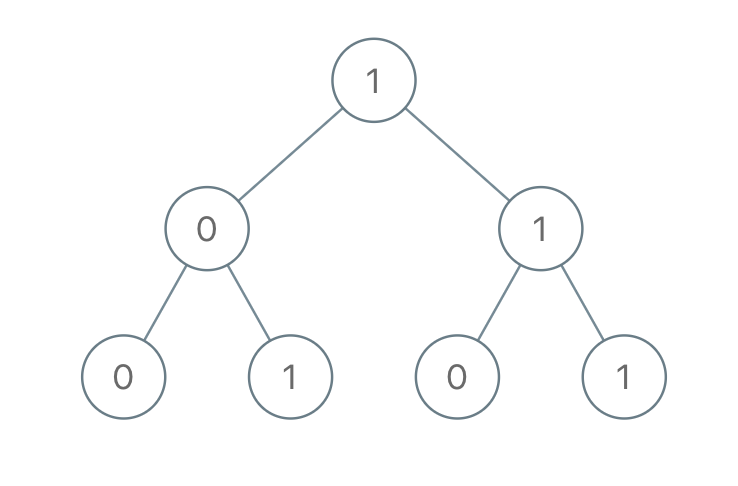# 1022. Sum of Root To Leaf Binary Numbers

Easy
You are given the `root` of a binary tree where each node has a value `0` or `1`. Each root-to-leaf path represents a binary number starting with the most significant bit.
• For example, if the path is `0 -> 1 -> 1 -> 0 -> 1`, then this could represent `01101` in binary, which is `13`.
For all leaves in the tree, consider the numbers represented by the path from the root to that leaf. Return the sum of these numbers.
The test cases are generated so that the answer fits in a 32-bits integer.
Example 1:Input: root = [1,0,1,0,1,0,1]
Output:
22
Explanation:
(100) + (101) + (110) + (111) = 4 + 5 + 6 + 7 = 22
Example 2:
Input: root = 
Output:
0
Constraints:
• The number of nodes in the tree is in the range `[1, 1000]`.
• `Node.val` is `0` or `1`.

### 解題

/**
* Definition for a binary tree node.
* type TreeNode struct {
* Val int
* Left *TreeNode
* Right *TreeNode
* }
*/
func sumRootToLeaf(root *TreeNode) int {
sum := 0
var recursive func(*TreeNode, string)
recursive = func(root *TreeNode, str string) {
if root.Left==nil && root.Right==nil {
sum += convert(str)
}
if root.Left!=nil {
recursive(root.Left, str+strconv.Itoa(root.Left.Val))
}
if root.Right!=nil {
recursive(root.Right, str+strconv.Itoa(root.Right.Val))
}
}
recursive(root, strconv.Itoa(root.Val))
// fmt.Println(sum)
return sum
}
func convert(str string) int {
if i, err := strconv.ParseInt(str, 2, 64); err != nil {
fmt.Println(err)
} else {
return int(i)
}
return 0
}

func sumRootToLeaf(root *TreeNode) int {
return dfs(root, 0)
}
func dfs(root *TreeNode, currSum int) int {
currSum = (currSum << 1) | root.Val
if root.Left == nil && root.Right == nil {
return currSum
}
total := 0
if root.Left != nil {
total += dfs(root.Left, currSum)
}
if root.Right != nil {
total += dfs(root.Right, currSum)
}
}

Runtime: 0 ms, faster than 100.00%
Memory Usage: 3.1 MB, less than 54.76%
/**
* Definition for a binary tree node.
* type TreeNode struct {
* Val int
* Left *TreeNode
* Right *TreeNode
* }
*/
func sumRootToLeaf(root *TreeNode) int {
sum := 0
var recursive func(*TreeNode, int)
recursive = func(root *TreeNode, currSum int) {
currSum = (currSum << 1) | root.Val
if root.Left==nil && root.Right==nil {
sum += currSum
}
if root.Left!=nil {
recursive(root.Left, currSum)
}
if root.Right!=nil {
recursive(root.Right, currSum)
}
}
recursive(root, 0)
return sum
}